The plot_bar_category() to visualizes the distribution of categorical data by level or relationship to specific numerical data by level.

plot_bar_category(.data, ...)

# S3 method for data.frame
plot_bar_category(
.data,
...,
top = 10,
title = "Frequency by levels of category",
each = FALSE,
typographic = TRUE,
base_family = NULL
)

# S3 method for grouped_df
plot_bar_category(
.data,
...,
top = 10,
title = "Frequency by levels of category",
each = FALSE,
typographic = TRUE,
base_family = NULL
)

## Arguments

.data a data.frame or a tbl_df or a grouped_df. one or more unquoted expressions separated by commas. You can treat variable names like they are positions. Positive values select variables; negative values to drop variables. If the first expression is negative, plot_bar_category() will automatically start with all variables. These arguments are automatically quoted and evaluated in a context where column names represent column positions. They support unquoting and splicing. an integer. Specifies the upper top rank to extract. Default is 10. logical. Decide whether to include text variables in the diagnosis of categorical data. The default value is TRUE, which also includes character variables. character. a main title for the plot. logical. Specifies whether to draw multiple plots on one screen. The default is FALSE, which draws multiple plots on one screen. logical. Whether to apply focuses on typographic elements to ggplot2 visualization. The default is TRUE. if TRUE provides a base theme that focuses on typographic elements using hrbrthemes package. character. The name of the base font family to use for the visualization. If not specified, the font defined in dlookr is applied. (See details)

## Details

The distribution of categorical variables can be understood by comparing the frequency of each level. The frequency table helps with this. As a visualization method, a bar graph can help you understand the distribution of categorical data more easily than a frequency table.

The base_family is selected from "Roboto Condensed", "Liberation Sans Narrow", "NanumSquare", "Noto Sans Korean". If you want to use a different font, use it after loading the Google font with import_google_font().

## Examples

# Generate data for the example
# \donttest{
heartfailure2 <- heartfailure
heartfailure2[sample(seq(NROW(heartfailure2)), 20), "platelets"] <- NA
heartfailure2[sample(seq(NROW(heartfailure2)), 5), "smoking"] <- NA

set.seed(123)
heartfailure2$test <- sample(LETTERS[1:15], 299, replace = TRUE) heartfailure2$test[1:30] <- NA

# Visualization of all numerical variables
plot_bar_category(heartfailure2)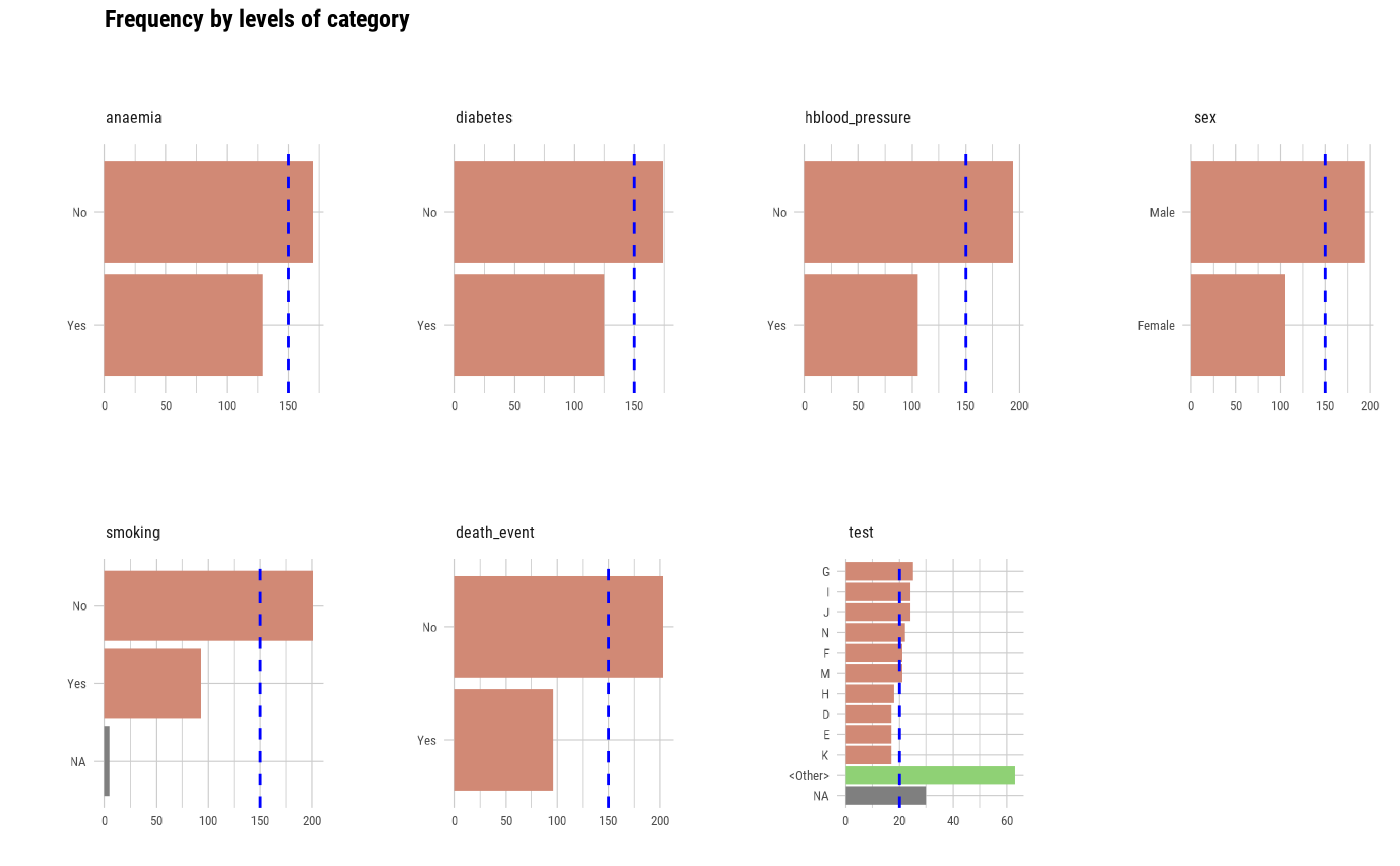# Select the variable to diagnose
plot_bar_category(heartfailure2, "test", "smoking")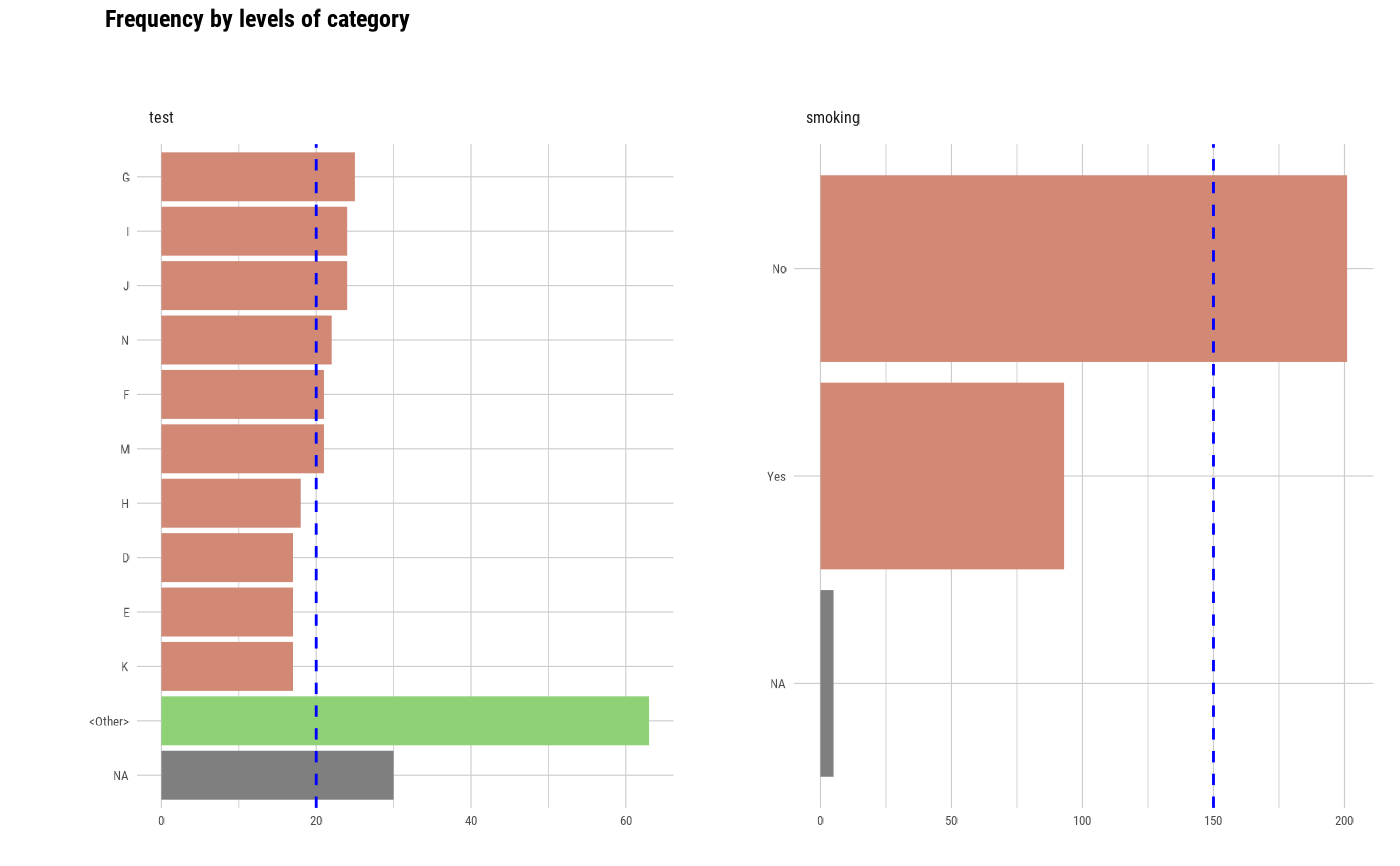plot_bar_category(heartfailure2, -test, -smoking)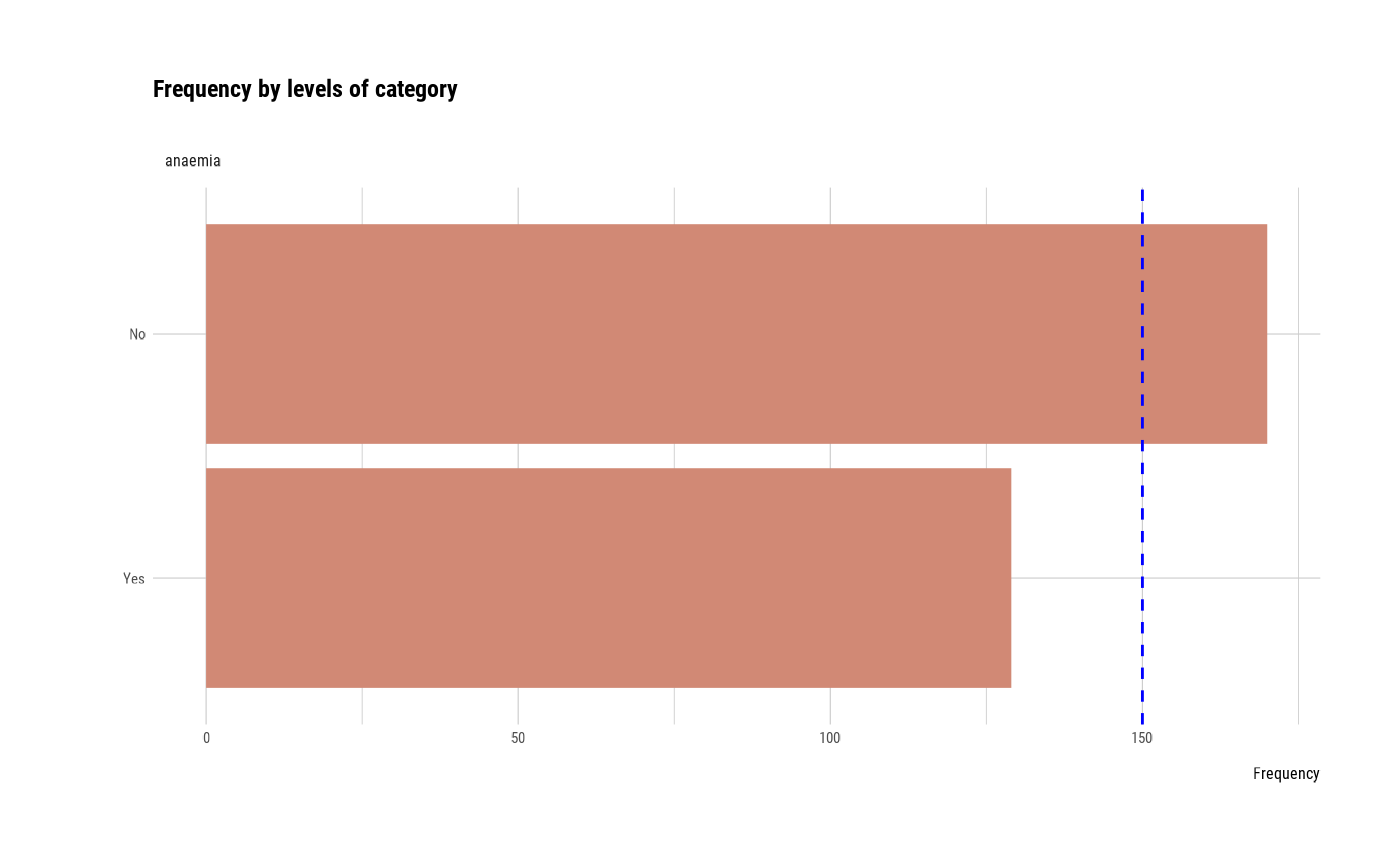# Visualize the each plots
plot_bar_category(heartfailure2, each = TRUE)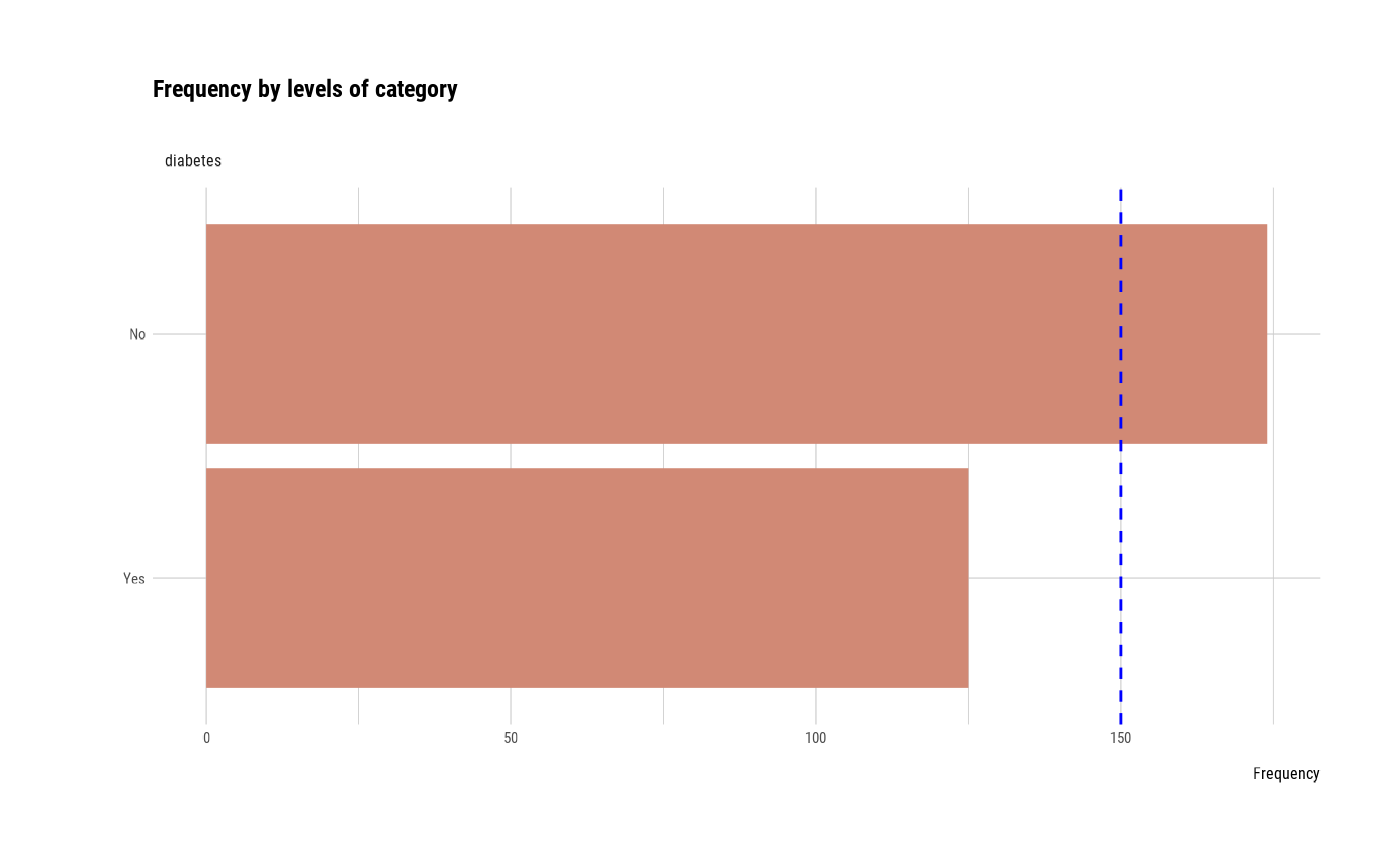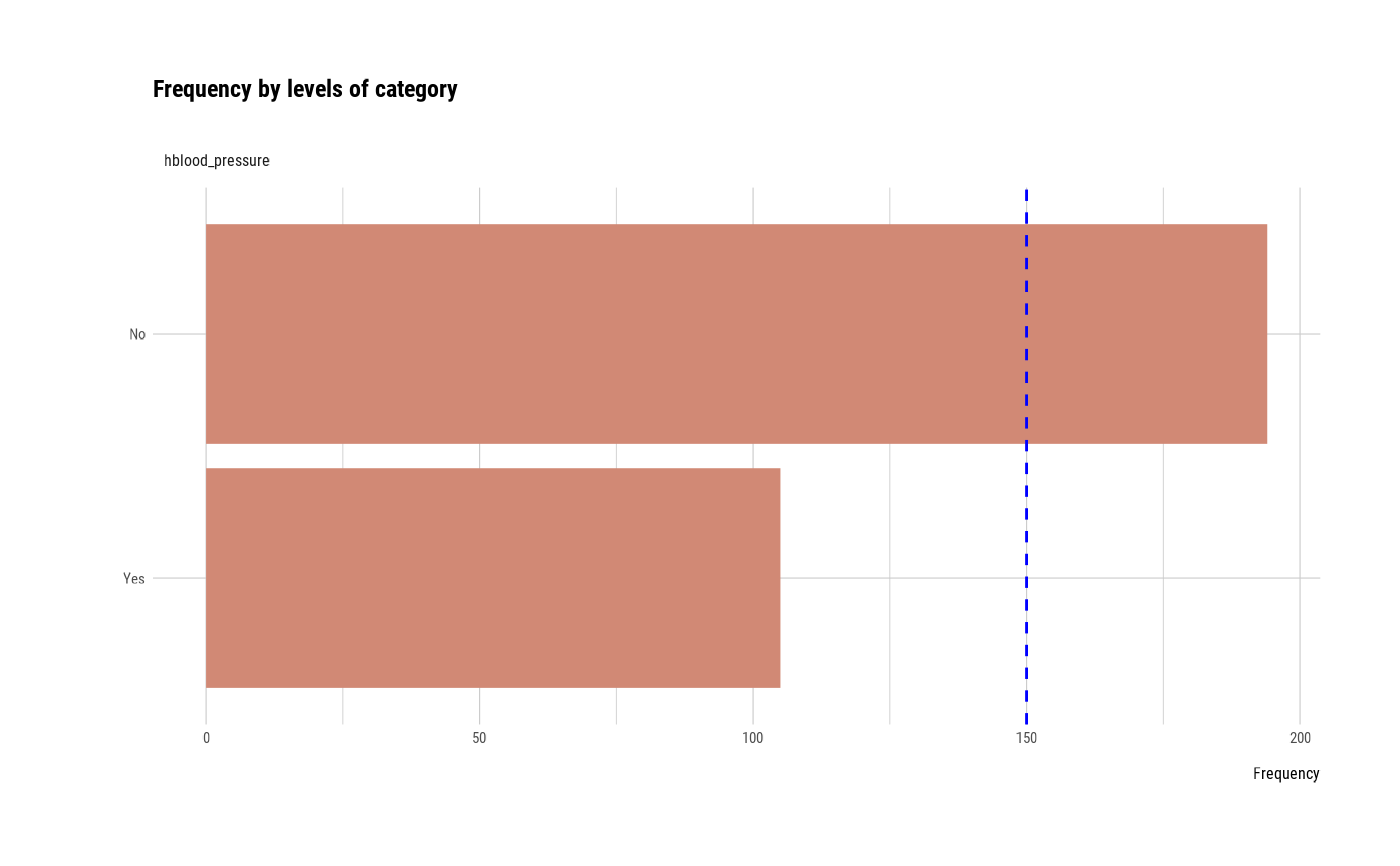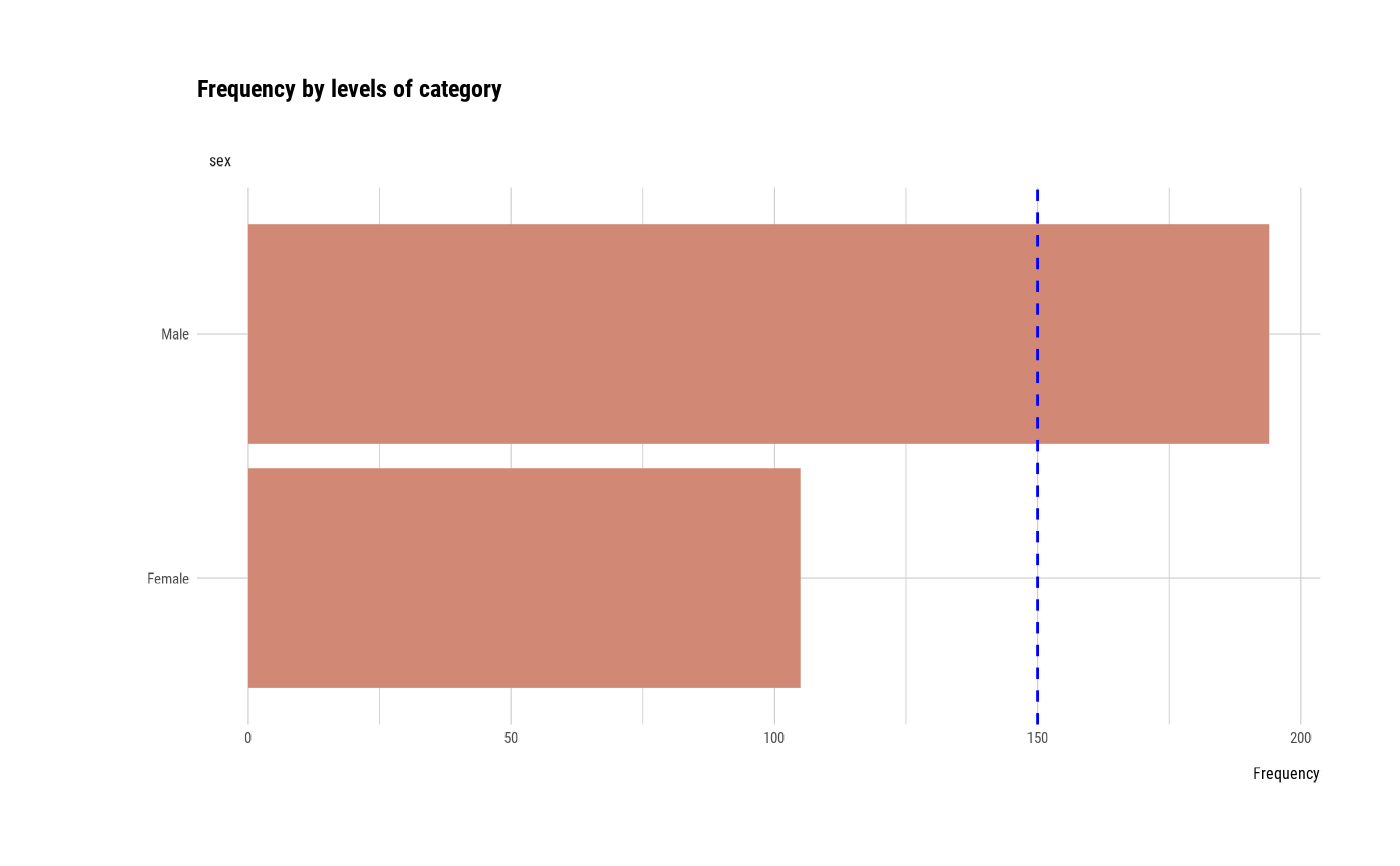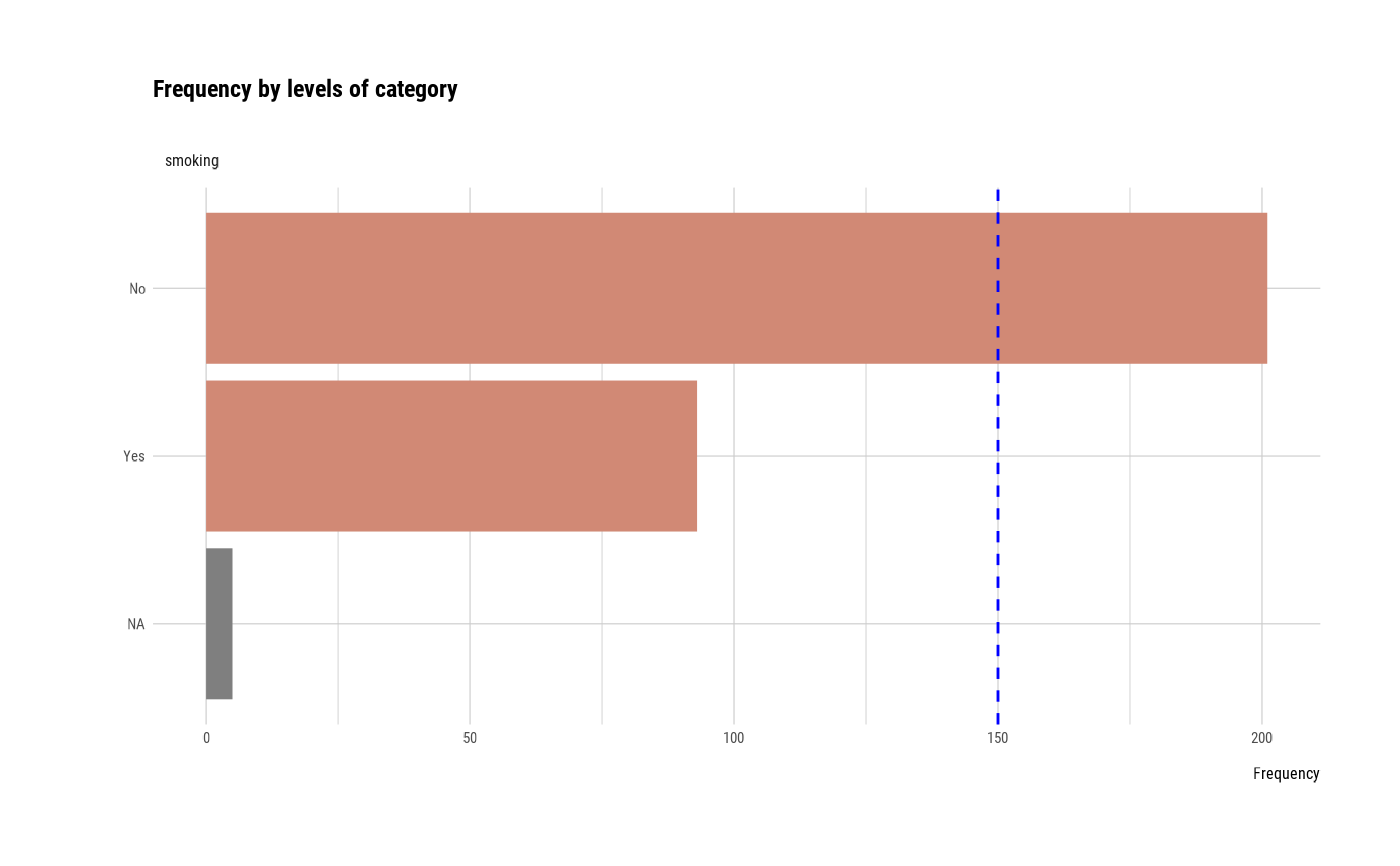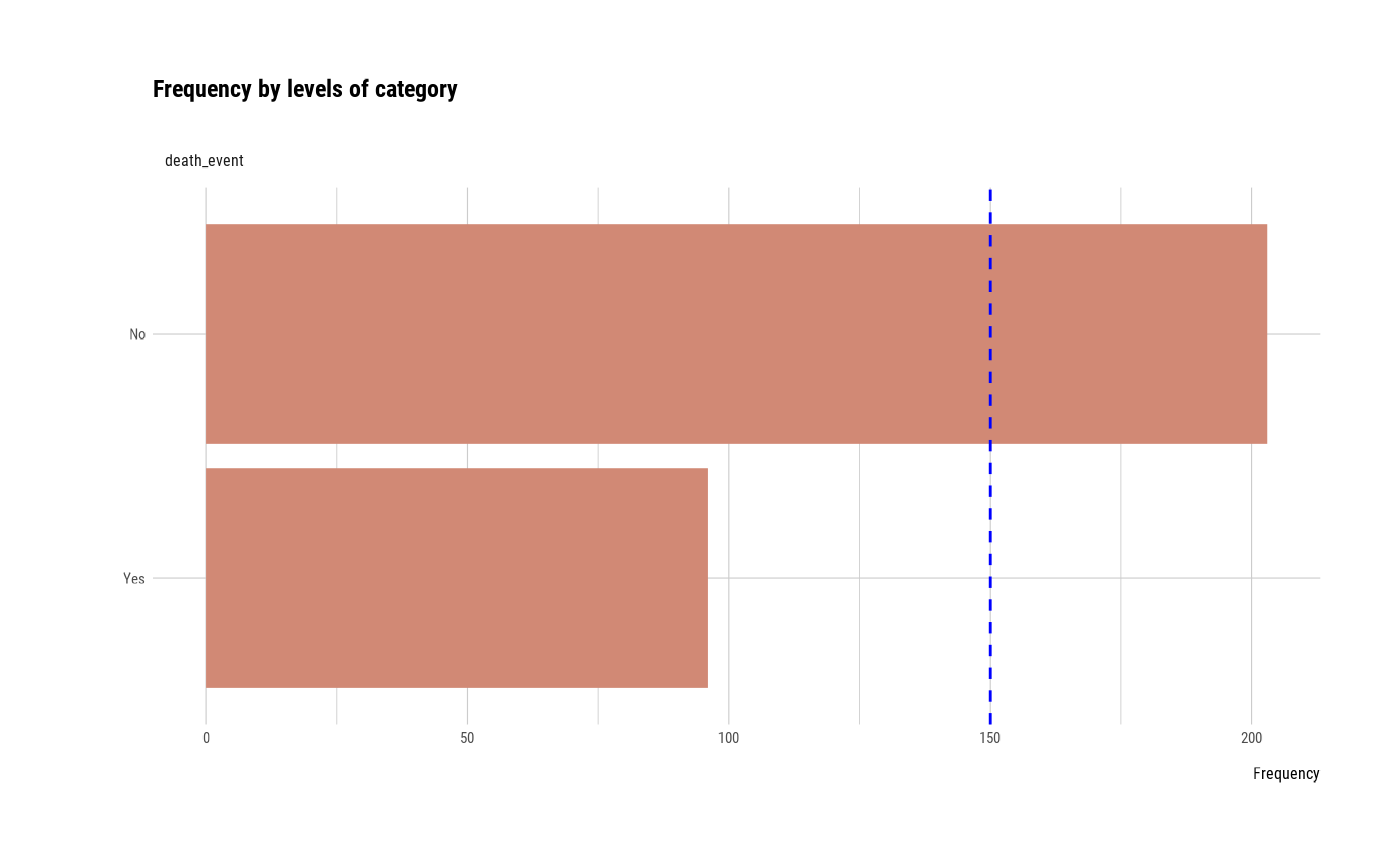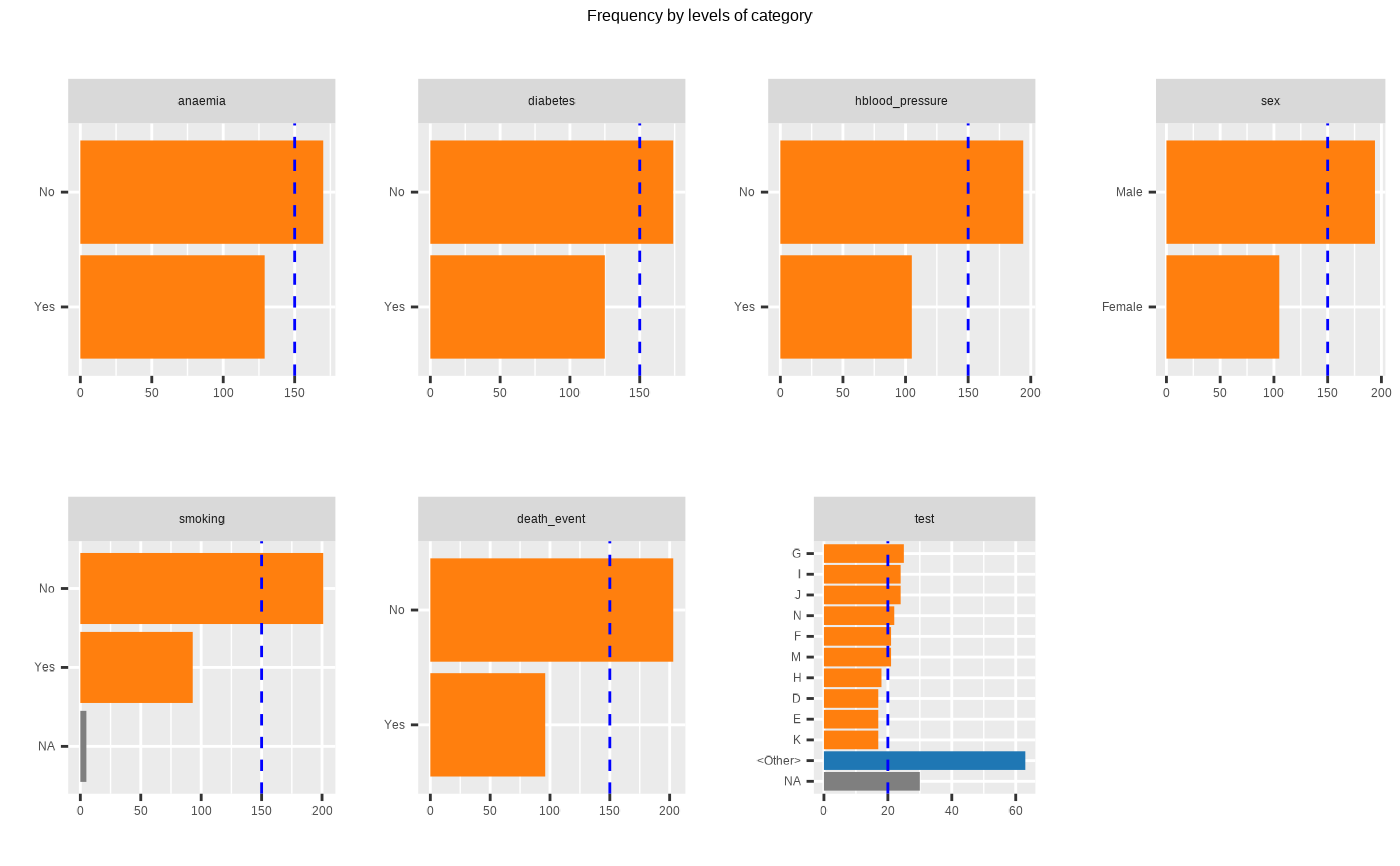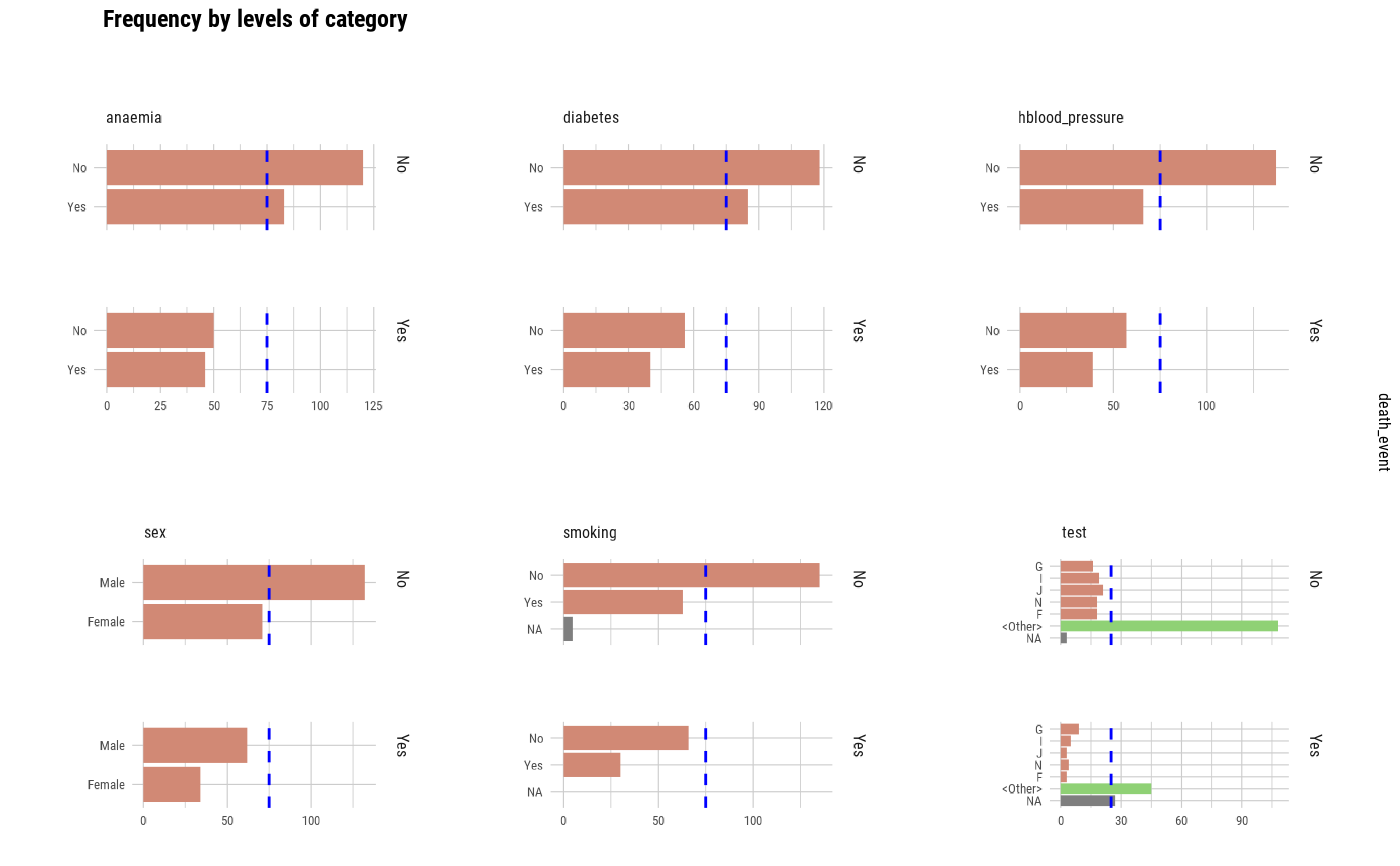# Not allow typographic argument
plot_bar_category(heartfailure2, typographic = FALSE)# Using pipes ---------------------------------
library(dplyr)

# Plot of all categorical variables
heartfailure2 %>%
plot_bar_category()# Visualize just 7 levels of top frequency
heartfailure2 %>%
plot_bar_category(top = 7)# Visualize only factor, not character
heartfailure2 %>%
plot_bar_category(add_character = FALSE)# Using groupd_df  ------------------------------
heartfailure2 %>%
group_by(death_event) %>%
plot_bar_category(top = 5)heartfailure2 %>%
group_by(death_event) %>%
plot_bar_category(each = TRUE, top = 5)# }